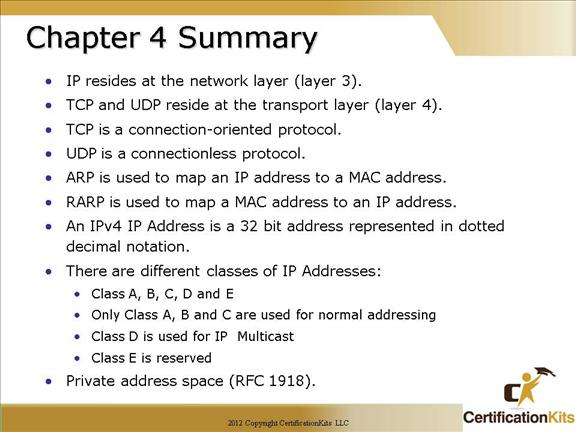Cisco CCNA Easy Subnetting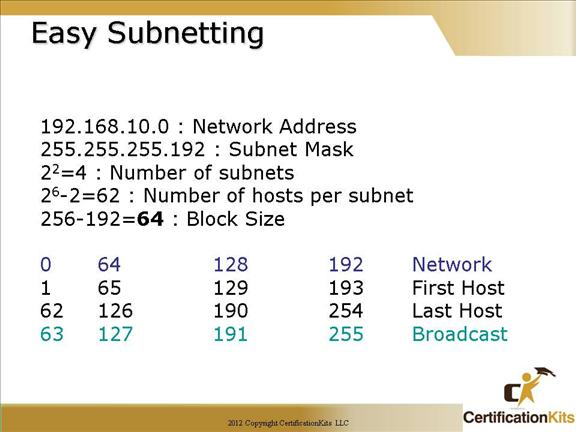192.168.10.0 = Network address

255.255.255.192 = Subnet mask

How many subnets?

Since 192 is two bits on (11000000), the answer would be 22 = 4.

How many hosts per subnet? We have 6 host bits off (11000000), so the equation would be 26 – 2 = 62 hosts.

What are the valid subnets? 256 – 192 = 64, which gives us our block size.  Start with 0 and keep adding the block size to get our valid subnets.

Our valid subnets are, then, 0, 64, 128 and 192.

Whats the broadcast address for each subnet? The number right before the next subnet is all host bits turned on and equals the broadcast address.

What are the valid hosts? These are the numbers between the subnets and the broadcast address. The easiest way to find the hosts is to write out the subnet address and the broadcast address. This way the valid hosts are obvious.

Cisco CCNA Now Implement it….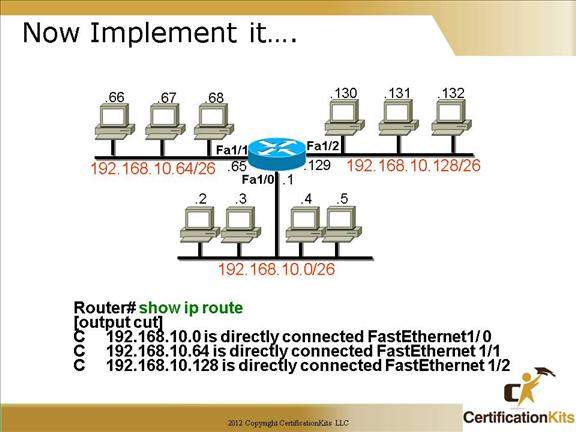This slide shows the output of a “show ip route” command when a Class C network is subnetted to a /26 (255.255.255.192) mask.

The routers can use any valid host address in the subnet, the hosts just need to know what it is so they can set the default gateway to that address. Each subnet supports 62 addresses per subnet, 64 total addresses (equals block size) where the first address is for the network and the last address is the broadcast address.

Cisco CCNA Easy Subnetting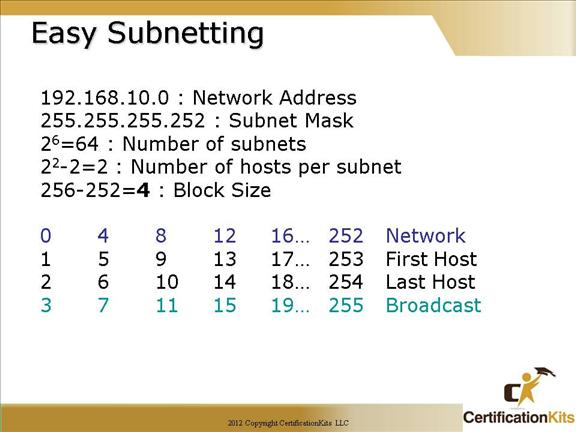192.168.10.0 = Network address

255.255.255.252 = Subnet mask

Subnets? 64.

Hosts? 2.

Valid subnets? 256 – 252 = 4 which gives us our block size. Start with 0 and keep adding the block size to get our  valid subnets.

0, 4, 8, 12, etc., all the way to 252.

Broadcast address for each subnet? Always the number right before the next subnet.

Valid hosts? The numbers between the subnet number and the broadcast address.

Cisco CCNA Now Implement it….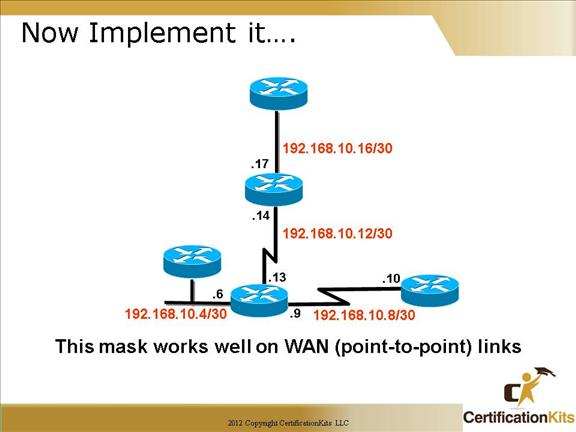It is very common to use 255.255.255.252 or /30 bit mask on point-to-point links.  Although you can use a larger range, since there is only two possible hosts on a point-to-point link, it makes sense to assign a /30 network to it.  Doing this we can save on valuable IP addresses. Each subnet supports 2 addresses per subnet, 4 total addresses (equals block size) where the first address is for the network and the last address is the broadcast address.

Cisco CCNA Easy Subnetting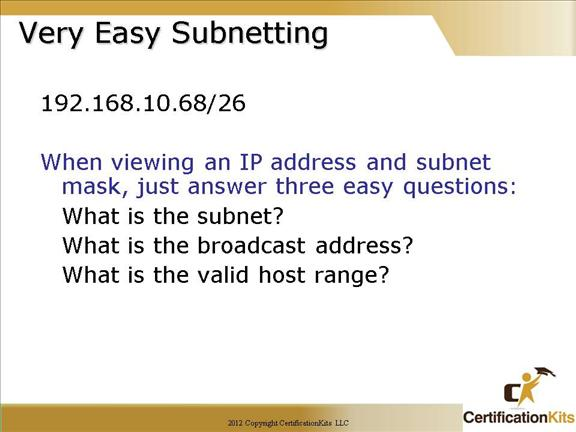192.168.10.68          255.255.255.192

192.168.10.64          Subnet

192.168.10.65          First Host

192.168.10.126        Last Host

Cisco CCNA Subnet Question255.255.255.0 = 254 hosts per subnet

255.255.255.128 = 126 hosts per subnet

255.255.255.192 = 62 hosts per subnet – Correct Answer

255.255.255.224 = 30 hosts per subnet

255.255.255.240 = 14 hosts per subnet

255.255.255.248 = 6 hosts per subnet

255.255.255.252 = 2 hosts per subnet

Always round up.  It can be quite difficult to change the subnet mask after you have assigned adjacent address space, or if the expanded range starts on another address.  Although it is a good practice to conserve address space wherever possible, it may become necessary to allocate, reserve or pre-assign address space based on a capacity requirement, potential, or perceived need.  Note: You will need to run a classless routing protocol in order to subnet address space utilizing VLSM.

Cisco CCNA Subnet Question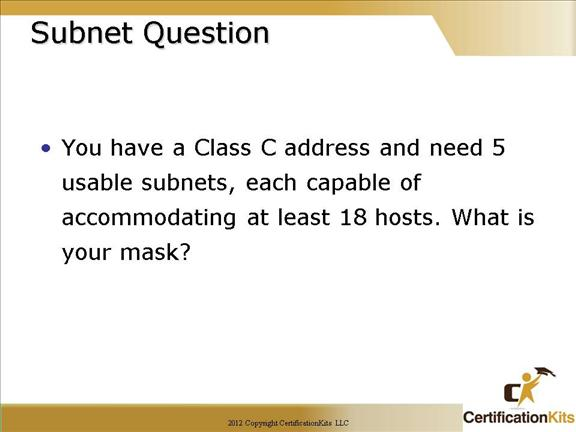255.255.255.0 = 254 hosts per subnet

255.255.255.128 = 126 hosts per subnet

255.255.255.192 = 62 hosts per subnet

255.255.255.224 = 30 hosts per subnet (Correct answer)

255.255.255.240 = 14 hosts per subnet

255.255.255.248 = 6 hosts per subnet

255.255.255.252 = 2 hosts per subnet

If the Class C address space is 192.168.0.0/24 then your subnets will be as follows:

192.168.0.0/27

192.168.0.32/27

192.168.0.64/27

192.168.0.96/27

192.168.0.128/27

Cisco CCNA Subnet Question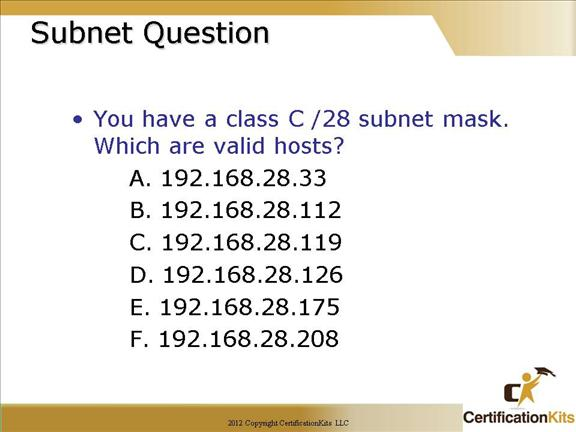As with the previous question, it is easier then it looks. We are subnetting only in the fourth octet.

Since this is a 240 mask (block size of 16) our valid subnets are 0, 16, 32, 48, 64,  80, 96, 112, 128, 144, 160, 176, 192, 208, 224 and 240.

• • Is a valid host
• • Is a subnet address
• • Is a valid host
• • Is a valid host
• • Is a subnet address

Cisco CCNA What is the host IP and default gateway address?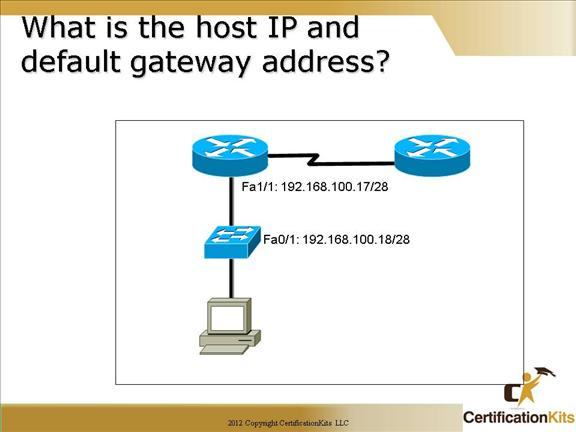The host address can be any unused IP address in the range. A /28 mask is a block size of 16, so the subnets are 0, 16, 32, 48…The routers interface is in the 16 subnet, so the host range is 17-30.

The default gateway configured on the host would be the routers Fa1/1 interface address which is 192.168.100.17.   Remember, switches are layer 2 devices by default (although a lot of switches these days are layer 3 capable).  In this case we are treating the switch as a layer 2 device so the default gateway needs to be an address on a layer 3 device which is the Fa1/1 interface of the router.

Cisco CCNA Chapter 4 Summary